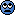A Determinism: mathematical models

Auto-Didact

Ever since Newton, determinism - i.e. the concept that the prior state of a system determines the next state of the system - has become an integral part of science, in particular of physics. This is particularly because Newton invented a mathematization of the concept of change: the calculus; in doing so he did much more.

Applying this new form of mathematics to study changes of physical phenomenon, Newton discovered that the concept of motion follows very strict mathematical laws; this essentially was the discovery of the laws of physics. The laws of physics have since then always come to us in the form of differential equations (or generalizations thereof).

To make a long story short, differential equations - or any abstractions thereof or of descriptive functions where the input and outputs reside in the same space - are the prototypical mathematical implementation (or 'mathematization') of the concept of determinism.

My question is, is anyone familiar with other forms of determinism which can definitively not be reduced to or be shown to be equivalent to differential equations in any sense, not even in principle?

Related Other Physics Topics News on Phys.org

hilbert2

Gold Member
If we have a discrete-time system, where the time variable jumps forward in constant steps, then it would be a difference equation.

A system with memory effects, like a particle acted on by a force field that depends on where the particle was 1 second ago and not on its current position, is not described by a differential equation (if a DE is defined in the usual way). It can be formally written like a DE that has time derivatives of arbitrarily high order in it.

S.G. Janssens

My question is, is anyone familiar with other forms of determinism which can definitively not be reduced to or be shown to be equivalent to differential equations in any sense, not even in principle?
(Deterministic) cellular automata?

A system with memory effects, like a particle acted on by a force field that depends on where the particle was 1 second ago and not on its current position, is not described by a differential equation (if a DE is defined in the usual way). It can be formally written like a DE that has time derivatives of arbitrarily high order in it.
It can also be written (rigorously, not just formally) as a DE in a Banach space (of infinite dimension).

•hilbert2 and Auto-Didact

Auto-Didact

If we have a discrete-time system, where the time variable jumps forward in constant steps, then it would be a difference equation.

...if a DE is defined in the usual way
I am not defining DEs in the usual way, but instead including all similar concepts such as difference equations, recurrence relations, iterative maps, dynamical systems, and other commonly employed mathematical abstractions used in physics and applied mathematics.
(Deterministic) cellular automata?
This is more in line with what I'm envisioning, but as far as I can tell deterministic cellular automata are outputs which function by producing more outputs based on inputs of their local environment. In other words, they are themselves solutions to some functions representative of what I'm arbitrarily calling DEs.

FactChecker

Gold Member
2018 Award
What about random processes whose result at some stage has probability one? Or whose average approaches some other process as a limit (e.g. the Central Limit Theorem)?

•Auto-Didact

OCR

If we have a discrete-time system. . .

Well, we might . . . but how would you ever know (measure it?) ??

. . .where the time variable jumps forward in constant steps. . .

That makes me think about the guy with the arrow. . . ..

sophiecentaur

Gold Member
Is it relevant to bring in Chaos here?

•Auto-Didact

Auto-Didact

What about random processes whose result at some stage has probability one? Or whose average approaches some other process as a limit (e.g. the Central Limit Theorem)?
Elaborate?
Is it relevant to bring in Chaos here?
As much as I love chaos, I'm afraid it isn't.

What I'm looking for is some other mathematical description of determinism, which cannot be reduced to being equivalent to DEs, or else a proof that such another mathematical description is impossible, i.e. that the class of deterministic conceptualizations = the class of objects which fall under my non-standard DE moniker.

•sophiecentaur

FactChecker

Gold Member
2018 Award
FactChecker said:
What about random processes whose result at some stage has probability one? Or whose average approaches some other process as a limit (e.g. the Central Limit Theorem)?
Elaborate?
I'm not sure that I can give a formal description. There are things whose state at particular times is determined (or approximately determined in the limit) even though the intermediate states are not deterministic. Do they fit into your original question?

Auto-Didact

I'm not sure that I can give a formal description. There are things whose state at particular times is determined (or approximately determined in the limit) even though the intermediate states are not deterministic. Do they fit into your original question?
They might. If I understand correctly you are saying that some aspects of outcomes based on probability theory and its theorems are in advance determined i.e. deterministic in some more abstract sense than determinism as usually understood.

Stated in this way I would I agree with this. The next question is, is the underlying reason for this more abstract determinism analogous or reducible to my non-standard DE definition or not?

•FactChecker

"Determinism: mathematical models"

Physics Forums Values

We Value Quality
• Topics based on mainstream science
• Proper English grammar and spelling
We Value Civility
• Positive and compassionate attitudes
• Patience while debating
We Value Productivity
• Disciplined to remain on-topic
• Recognition of own weaknesses
• Solo and co-op problem solving